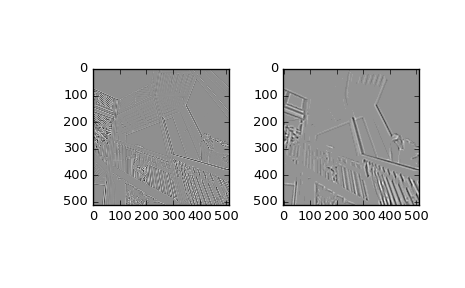# scipy.ndimage.gaussian_laplace¶

scipy.ndimage.gaussian_laplace(input, sigma, output=None, mode='reflect', cval=0.0, **kwargs)[source]

Multidimensional Laplace filter using gaussian second derivatives.

Parameters: input : array_like Input array to filter. sigma : scalar or sequence of scalars The standard deviations of the Gaussian filter are given for each axis as a sequence, or as a single number, in which case it is equal for all axes. output : array, optional The output parameter passes an array in which to store the filter output. mode : {‘reflect’, ‘constant’, ‘nearest’, ‘mirror’, ‘wrap’}, optional The mode parameter determines how the array borders are handled, where cval is the value when mode is equal to ‘constant’. Default is ‘reflect’ cval : scalar, optional Value to fill past edges of input if mode is ‘constant’. Default is 0.0 Extra keyword arguments will be passed to gaussian_filter().

Examples

>>> from scipy import ndimage, misc
>>> import matplotlib.pyplot as plt
>>> ascent = misc.ascent()

>>> fig = plt.figure()
>>> plt.gray()  # show the filtered result in grayscale
>>> ax1 = fig.add_subplot(121)  # left side
>>> ax2 = fig.add_subplot(122)  # right side

>>> result = ndimage.gaussian_laplace(ascent, sigma=1)
>>> ax1.imshow(result)

>>> result = ndimage.gaussian_laplace(ascent, sigma=3)
>>> ax2.imshow(result)
>>> plt.show()